OMB+
Learning Mathematics
What and How?
To the course
Only available in German
Registration

# Online Mathematics Bridging Course OMB+

„My time in school is a few years back. Before entering a physics study this was perfect to restore my math skills. Thanks and keep it up! “ (Philip, September 2016)
„The course is really super. It prepares us in my opinion very well and I feel much safer. Thank you for your effort and that one has always reached someone.“ (Rebecca, August 2016)
„ Thanks for this great preparatory course! The course is very vivid, almost entertaining (diagrams, videos, ...). Thank you, you made it that I really enjoy math!! “ (Nadine, September 2016)
„Overall, I find the course very pleasantly designed, almost everything was understandable on the first or second glance and the speedy help from the tutors has covered the rest properly.“ (Timo, October 2015)
Want to prepare yourself for a degree in engineering, economics, science or computer science? The online course OMB+ is made for just that. You will be able to get assistance in our mathematics call center using chat or the forum. The course helps you to refresh your high school mathematics knowledge so that you can easily follow freshmen German university courses.

ⅠA Elementary Calculus: Sets and Numbers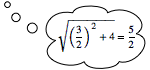ⅠB Elementary Calculus: Proportionality, Percentage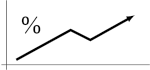Ⅱ Equations in One UnknownⅢ Inequalities in One VariableⅣ Linear Systems of Equations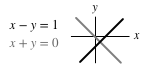Ⅴ GeometryⅥ Elementary FunctionsⅦ Differential CalculusⅧ Integral CalculusⅨ orientation in the 2 dimensional coordinate systemⅩ basics of intuitive vector geometry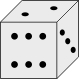Ⅺ Complex NumbersⅫ Logic and Sets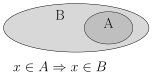## How is the course structured?

The main part of the course is topically divided into 11 chapters, contentwise based on
the recommendations of the COSH group, and additional modules exceeding those recommandations. Each chapter or additional module begins with an overview of its sections. A section consists of four components:

• Expository texts with built-in videos, explanations, examples, and quick checks of understanding: All important concepts are briefly explained in full.
• Exercises − This is how it's done: In sample problems the usual solution methods are shown in full.
• Training − Learning by doing: Here you can practice until you feel confident.
• Quiz − Understood everything?: This is where your understanding of the content is checked.

Each chapter or additional module has a final exam which checks your understanding of the content and concepts of its sections.

## Certificate

You will get a certificate for the successful completion of the course if you have achieved at least 80% of the maximum score in each final exams of all 11 chapters of the main course part. The certificate is recognized at some universities as course achievement.
Feedback
If you have encountered any problems or just want to get in touch with your opinion, then you can contact us here.
E-Mail :
Support

# How to type mathematics in the course?

The following mathematical operators can be used to create expressions:

OperatorExampleResult
Addition2+3$2+3$
Subtraction2-3$2-3$
Multiplication2*3$2\cdot 3$
Division2/3x+1, 2/(3x+1)$\frac{2}{3x}+1$, $\frac{2}{3x+1}$
Powers2^3, 2^(-3), (2^3)^4${2}^{3}$, ${2}^{-3}$, $\left({2}^{3}{\right)}^{4}$
Rootssqrt(4), cbrt(9)$\sqrt{4}$, $\sqrt{9}$
Functionsexp(3x), e^(3x)$\mathrm{exp}\left(3x\right)$, ${e}^{3x}$
log(2), ln(e), log_5(25)$\mathrm{log}\left(2\right)$, $\mathrm{ln}\left(e\right)$, ${\mathrm{log}}_{5}25$
sin(2*pi), cos(2*pi), tan(2*pi)$\mathrm{sin}\left(2\pi \right)$, $\mathrm{cos}\left(2\pi \right)$, $\mathrm{tan}\left(2\pi \right)$
arcsin(pi), arccos(pi), arctan(pi)$\mathrm{arcsin}\left(\pi \right)$, $\mathrm{arccos}\left(\pi \right)$, $\mathrm{arctan}\left(\pi \right)$
Infinity-infty, infinity, +unendlich$-\infty$, $\infty$, $+\infty$
Info
In the OMB+ Chat and Forum you can also write mathematical expressions nicely, but they have to be wrapped in backticks. Both the Chat and Forum provide a button to insert these automatically.
Example: 1/sin(x) becomes 1/sin(x)

# Mathematics Call Center

Daily from 10:00 till 20:00 - also in weekends.
OMB+ ChatOMB+ Chat
Telephone +49 30 5771 4045
Forum Forum 
 The forum is in German only.If you have any questions regarding the course, need help solving a particular problem or would like more information regarding certain mathematical concepts, then we (the tutors) are happy to help.

Work on the course regularly for one long, continuous period of time. For example, four hours a day, from Monday to Friday, over the course of three weeks.

Try not using a calculator. This way you will benefit more from the course. For the goals of this course try only using them only in exceptional cases, for example for calculating $\mathrm{sin}\left(10\right)$ or $\mathrm{log}\left(3\right)$.

Even though the course can be worked on from a mobile device, to work on it comfortably we recommend to use a laptop or desktop computer

### We recommend that you work through the course in the following fashion:

Begin with Chapter I "Elementary Calculus" and work through the chapters in the given order (I, II, … ,X), as subsequent chapters may require knowledge from previous chapters.

#### Working on a chapter

A chapter consists of multiple sections and each section contains
• an article,
• practise exercises,
• traning exercises and
• a quiz.

For each section, you start working through the article so that you become familiar with all terms and concepts corresponding to the topic. Articles will come with visualizations, videos and quick-checks in order to help you understand the content better.

Once you are comfortable with all the terms and concepts from the article, you can do the practise exercises, strengthen your knowledge with the training exercises and finally test your knowledge in the quiz.

After successfully completing the quiz you continue to the next section.

#### When you are stuck at finding the solution of a problem

• First, try solving the solution yourself. If you succeed in this, the learning effect will be greater. To try this, first formulate the problem in your own words and then ask the following questions:
1. "Have I seen a similar problem before?"
2. "Can I solve a simpler version of the problem, or is there a special case that I can solve?"
3. "Can I break down the problem into smaller parts and solve those parts?"
4. "Have I thought about everything and used all the information given to me in the problem statement?"
5. "What could the solution look like?"
• If these questions still have not helped, you can contact our call center. Our tutors are happy to help you out.

You should only do the final exam of a chapter when you have understood the corresponding content. After successfully completing the final exam you can go to the next chapter.

OMB+ Chat
In order to use the chat functionality you should be logged in.